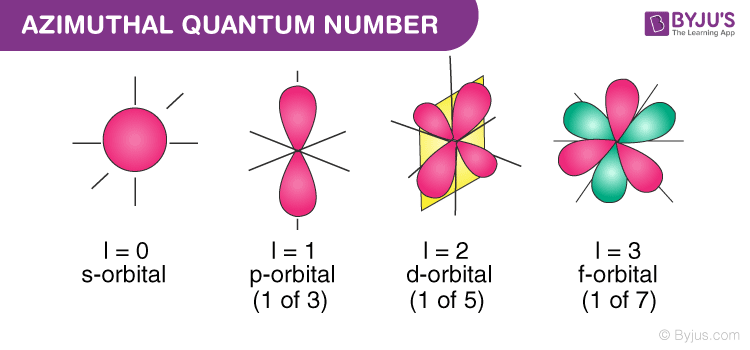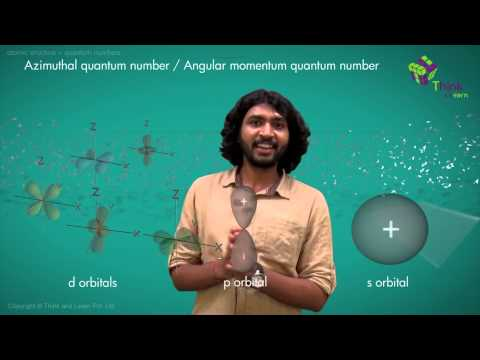Checkout JEE MAINS 2022 Question Paper Analysis : Checkout JEE MAINS 2022 Question Paper Analysis :

# Azimuthal Quantum Number

Quantum numbers are numbers allocated to all the electrons in an atom and they describe certain characteristics of the electron. The characteristics of the orbital are used to define the state of an electron completely and are expressed in terms of four numbers: principal quantum number, azimuthal quantum number, magnetic quantum number and spin quantum number. In this piece of article, we will be discussing azimuthal quantum numbers.

Table of Content

## What is Azimuthal Quantum Number?Other than principal quantum number (n), spectroscopic notation, magnetic quantum number (m) and the spin quantum number (s), the azimuthal quantum number is another set of quantum numbers which describe the unique quantum state of an electron. It can be defined as,

The quantum number is associated with the angular momentum of an atomic electron.

It is also termed the orbital angular momentum quantum number, orbital quantum number or second quantum number, and is symbolised as ℓ. This number describes the shape of the orbital and also determines the orbital angular momentum. An example of the angular quantum momentum number would be a p orbital that is associated with an azimuthal quantum number equal to 1.

## History

Arnold Sommerfeld posited the term azimuthal quantum number from the Bohr model of the atom. The Rutherford-Bohr model depicts the atom as a small, positively charged nucleus surrounded by electrons that travel in circular orbits around the nucleus – similar in structure to the solar system, but with attraction provided by electrostatic forces rather than gravity.

The Bohr model has its existence from spectroscopic analysis of the atom in combination with the Rutherford atomic model. Angular momentum was found to be ‘0’ at the lowest level of quantum. Orbits with zero angular momentum were termed ‘pendulum’ orbits.

### Subsidiary Quantum Number

Azimuthal quantum number describes the shape of the orbital. It is denoted by ℓ. Values of ℓ are from zero to n-1.

For s-orbital, ℓ = 0

For p-orbital, ℓ = 1

For d-orbital, ℓ = 2

For f-orbital, ℓ = 3

With the help of the value of the azimuthal quantum number, we can determine the total number of energy sublevels in a given energy level.

### Angular Momentum Quantum Number

• Intrinsic (or spin) angular momentum quantum number, or simply spin quantum number
• Magnetic quantum number, related to the orbital momentum quantum number
• Total angular momentum quantum number.## Frequently Asked Questions – FAQs

### What is the range of azimuthal quantum numbers?

The range of azimuthal quantum numbers is between 0 to n-1.

### What are the values of the spin quantum number?

The values of the spin quantum number is are -½ or +½.

### For a quantum number, if n=4, what is the value that is not equal to l?

There are three quantum numbers: n, l, and m. The principal quantum number is n. According to the questions, the principal quantum number, n = 4. Therefore, l, which is the angular quantum number, can have values 0, 1, 2, 3 and not beyond 3.

### Name the quantum number that describes the size of the orbital.

The principal quantum number describes the size of the orbital. The principal quantum number cannot be zero, and the size of the orbit increases with an increase in numbers.

### What are the 4 types of quantum numbers?

The 4 types of quantum numbers are principal quantum number, angular momentum, magnetic moment, and spin quantum number.

Test your knowledge on Azimuthal Quantum Number